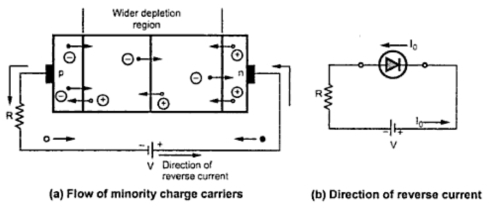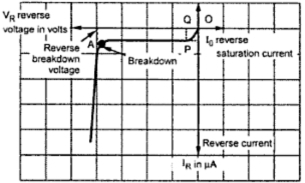### Reverse Biasing of P-N Junction Diode

If an external d.c. voltage is connected in such a way that the p-region terminal of a p-n junction is connected to the negative of the battery and the n-region terminal of a p-n junction is connected to the positive terminal of the battery, the biasing condition is called reverse biasing of a p-n junction.
Note : Reverse biasing means connecting p-region to -ve and n-region to +ve of the battery.
The Fig. 1(a) shows the connection of a reverse biasing of a p-n junction while the Fig. 1(b) shows the symbolic representation of a reverse biased diode.Fig. 1
1.1 Operation of Reverse Biased Diode
When the p-n junction is reverse biased the negative terminal attracts the holes in the p-region, away from the junction. The positive terminal attracts the free electrons in the n-region away from the junction. No charge carrier is able to cross the junction, the depletion region widens. This creates more positive ions and hence more positive charge in the n-region and more negative ions and hence more negative charge in the n-region. This is because the applied voltage helps the barrier potential. This is shown in the Fig. 2.Fig. 2  Depletion region widens in reverse bias
Note : Reverse biasing increases the width of the depletion region.
As depletion region widens, barrier potential across the junction also increases. However, this process can not continue for long time. In the steady state, majority current ceases as holes and electrons stop moving away from the junction.
The polarities of barrier potential are same as that of the applied voltage. Due to increased barrier potential, the positive side drags the electrons from p-region towards the positive of battery. Similarly negative side of barrier potential drags the holes from n-region towards the negative of battery. The electrons on p side and holes on n side are minority charge carriers, which constitute the current in reverse biased condition. Thus reverse conduction takes place.
The reverse current flows due to minority charge carriers which are small in number. Hence revers current is always very small.
Note : The generation of minority charge carriers depends on the temperature and not on the applied reverse bias voltage. Thus the reverse current depends on the temperature i.e. thermal generation and not on the reverse voltage applied.
For a constant temperature, the reverse current is almost constant though reverse voltage is increased upto a certain limit. Hence it is called reverse saturation current and denotes as  I0.
Note : Reverse saturation current is very small of the order of few microamperes for germanium and few nanoamperes for silicon p-n junction diodes.
The reverse current and its direction is shown in the Fig. 3.Fig. 3   Reverse biased diode
The reverse biasing produces a voltage drop across the diode denoted as which is almost equal to applied reverse voltage.
1.2 Breakdown in Reverse Biased
Though the reverse saturation current is not dependent on the applied reverse voltage, if reverse voltage is increased beyond particular value, large reverse current can flow damaging the diode. This is called reverse breakdown of a diode. Such a reverse breakdown of a diode can take place due to the following two effects.
1. Avalanche   effect and       2. Zener effect
1.2.1 Breakdown due to the Avalanche Effect
Though reverse current is not dependent on reverse voltage, if reverse voltage is increased, at a particular value, velocity of minority carriers increases. Due to the kinetic energy associated with the minority carriers, more minority carriers are generated when there is collision of minority carriers with the atom. The collision make the electrons to break the covalent bonds. These electrons are available as minority carriers and gey accelerated due to high reverse voltage. They again collide with another atoms to generate more minority carriers. This is called carrier multiplication. Finally large number of minority carriers move across the junction, breaking the p-n junction. These large number of minority carriers give rise to a very high reverse current. This effect is called avalanche effect and the mechanism of destroying the junction is called reverse breakdown of a p-n junction. The voltage at which the breakdown of a p-n junction occurs is called reverse breakdown voltage. The series resistance must be used to avoid breakdown condition, limiting the reverse current.
1.2.2 Breakdown due to the Zener Effect
The breakdown of a p-n junction may occur because of one more effect called zener effect. When a p-n junction is heavily doped the depletion region is very narrow. So under reverse bias conditions, the electric field across the depletion layer is very intense. Electric field is voltage per distance and due to narrow depletion region and high reverse voltage, it is intense. Such an intense field is enough to pull the electrons out of the valence bands of the stable atoms. So this is not due to the collision of carriers with atoms. Such a creation of free electrons is called zener effect which is different than the avalanche effect. These minority carriers constitute very large current and mechanism is called zener breakdown.
Why to avoid reverse breakdown?
1. Large reverse voltage appears across the diode and large current flows through the diode in reverse breakdown condition.
2. So large power gets dissipated which appears in the form of heat at the junction.
3. This increase junction temperature beyond the safe limits and this may damage the diode permanently.
So reverse breakdown must be avoided for conventional diodes.
Some special diodes are manufacturer to be operated in the reverse breakdown region and are called zener diodes.
1.3 Reverse Characteristics of P-N Junction Diode
The Fig. 4 shows the reverse biased diode. The reverse voltage across the diode is VR while the current flowing is reverse current IR flowing due to minority charge carriers. The graph of IR against VR is called reverse characteristics of a diode.Fig. 4  Reverse biased diode
The polarity of reverse voltage applied is opposite to that of forward voltage. Hence in practice reverse voltage is taken as negative. Similarly the reverse saturation current is due to the minority carriers and is opposite to the forward current. Hence in practice reverse saturation current is also taken as negative. Hence the reverse characteristics is plotted in the third quadrant as shown in the Fig. 5.Fig. 5
Note : Typically the reverse breakdown voltage is greater than 50 V for normal p-n junctions.
As reverse voltage is increaed, reverse current increases initially but after a certain voltage, the current remains constant equal to reverse saturation current thoug reverse voltage is increased. The point A where breakdown occurs and reverse current increases rapidly is called knee of the reverse characteristics.
1.4 Reverse Resistance of Diode
The p-n junction offers large resistance in the reverse biased condition called reverse resistance. his is also defined in two ways.
1. Reverse static resistance :
This is reverse resistance under d.c. conditions, denoted as Rr. It is the ratio of applied reverse voltage to the reverse saturation current I0.
...               Rr = OQ/I0 = Applied reverse voltage/Reverse saturation current
2. Reverse dynamic resistance :
This is the reverse resistance under the a.c. conditions, denoted as . It is the ratio of incremental change in the reverse voltage applied to the corresponding change in the reverse current.
...               rr = ΔVR /ΔIR = change reverse voltage/change in revers current
The dynamic resistance is most important in practice whether the junction is forward or reverse biased.